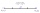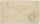Arithmetic average

The arithmetic mean of the five numbers is exactly 8. The sum of these four numbers is 30. What is the fifth number?

Result

x =  10

Solution:

(30+x)/5 = 8

x = 10

x = 10

Calculated by our simple equation calculator.

Leave us a comment of example and its solution (i.e. if it is still somewhat unclear...):Be the first to comment!To solve this example are needed these knowledge from mathematics:

Looking for help with calculating arithmetic mean? Looking for a statistical calculator? Do you have a linear equation or system of equations and looking for its solution? Or do you have quadratic equation?

Next similar examples:

1. AverageThe arithmetic mean of the two numbers is 71.7. One number is 5. Calculate the second number.
2. Find midpointFM=5y+13, MG=5-3y, FG=? M is the midpoint of FG. Use the given information to find the missing measure or value.
3. One-thirdA one-third of unknown number is equal to five times as great as the difference of the same unknown number and number 28. Determine the unknown number.
4. Dropped sheetsThree consecutive sheets dropped from the book. The sum of the numbers on the pages of the dropped sheets is 273. What number has the last page of the dropped sheets?
5. EquationSolve the equation: 1/2-2/8 = 1/10; Write the result as a decimal number.
6. Unknown numberIdentify unknown number which 1/5 is 40 greater than one tenth of that number.
7. Unknown number 11That number increased by three equals three times itself?
8. Negative in equation2x + 3 + 7x = – 24, what is the value of x?
9. Simple equationSolve the following simple equation: 2. (4x + 3) = 2-5. (1-x)
10. Equation 29Solve next equation: 2 ( 2x + 3 ) = 8 ( 1 - x) -5 ( x -2 )
11. Equation?
12. Simple equationSolve for x: 3(x + 2) = x - 18
13. Find xSolve: if 2(x-1)=14, then x= (solve an equation with one unknown)
14. NormThree workers planted 3555 seedlings of tomatoes in one dey. First worked at the standard norm, the second planted 120 seedlings more and the third 135 seedlings more than the first worker. How many seedlings were standard norm?
15. Forest nurseryIn the forest nursery after winter, they found that 1/10 stems died out of them. For them, they land 193 new spruces. How many spruces are in the forest nursery?
16. CakesGrandmother baked cakes. Half of its was poppy, quarter with plum jam and 16 cheesecakes. How many cakes she baked in total?
17. AuntAnnie aunt is 35 years old, Annie is 14 years. For how many years will aunt have twice as many years as Annie?